# Node.js http 伺服器建立

`Node.js官方網站 <http://Node.js.org>`裡面有舉一個最簡單的HTTP 伺服器建立，一開始初步就是建立一個伺服器平台，讓 Node.js 可以與瀏覽器互相行為。每種語言一開始的程式建立都是以 Hello world 開始，最初也從 Hello world 帶各位進入 Node.js 的世界。

``````var server,
ip   = "127.0.0.1",
port = 1337,
http = require('http');

server = http.createServer(function (req, res) {
res.end('Hello World\n');
});

server.listen(port, ip);

console.log("Server running at http://" + ip + ":" + port);
``````

• ip: 機器本身的ip 位置，因為使用本地端，因此設定為 127.0.0.1
• port: 需要開通的阜號，通常設定為 http port 80，因範例不希望與基本 port 相衝，隨意設定為 1337

``````res.writeHead(200, {'Content-Type': 'text/plain'});
res.end('Hello World\n');
``````

http 伺服器需要設定 port, ip，在最後需要設定 http 監聽，需要使用到 listen 事件，監聽所有 http 伺服器行為。

``````http.listen(port, ip);
``````

``````console.log("Server running at http://" + ip + ":" + port);
``````

# Node.js http 路徑建立

``````server = http.createServer(function (req, res) {
console.log(req.url);
res.end('hello world\n');
});
````````````var server,
ip   = "127.0.0.1",
port = 1337,
http = require('http'),
url = require('url'),
path;

server = http.createServer(function (req, res) {
path = url.parse(req.url);

switch (path.pathname) {
case "/index":
res.end('I am index.\n');
break;
case "/test":
res.end('this is test page.\n');
break;
default:
res.end('default page.\n');
break;
}
});

server.listen(port, ip);

console.log("Server running at http://" + ip + ":" + port);
``````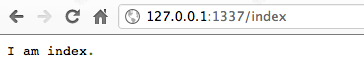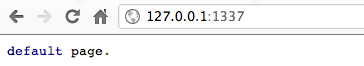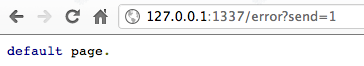# Node.js 檔案讀取

``````<!DOCTYPE html>
<html xmlns="http://www.w3.org/1999/xhtml" xml:lang="zh-tw" lang="zh-tw">
<meta http-equiv="Content-Type" content="text/html; charset=UTF-8" />
<title>Node.js index html file</title>
<body>
<h1>Node.js index html file</h1>
</body>
</html>
``````

``````var fs = require("fs"),
filename = "static/index.html",
encode = "utf8";

console.log(file);
});
``````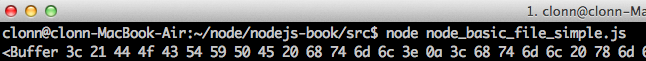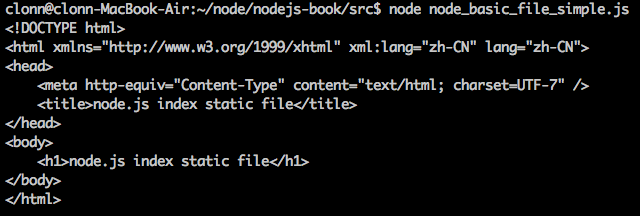# Node.js http 靜態檔案輸出

``````<!DOCTYPE html>
<html xmlns="http://www.w3.org/1999/xhtml" xml:lang="zh-TW" lang="zh-TW">
<meta http-equiv="Content-Type" content="text/html; charset=UTF-8" />
<title>Node.js index html file</title>
<body>
<h1>Node.js index html file</h1>
</body>
</html>
``````
``````<!DOCTYPE html>
<html xmlns="http://www.w3.org/1999/xhtml" xml:lang="zh-TW" lang="zh-TW">
<meta http-equiv="Content-Type" content="text/html; charset=UTF-8" />
<title>Node.js test html file</title>
<body>
<h1>Node.js test html file</h1>
</body>
</html>
``````
``````<!DOCTYPE html>
<html xmlns="http://www.w3.org/1999/xhtml" xml:lang="zh-TW" lang="zh-TW">
<meta http-equiv="Content-Type" content="text/html; charset=UTF-8" />
<title>Node.js static html file</title>
<body>
<h1>Node.js static html file</h1>
</body>
</html>
``````

``````var server,
ip   = "127.0.0.1",
port = 1337,
http = require('http'),
url = require('url');

server = http.createServer(function (req, res) {
var path = url.parse(req.url);
});

server.listen(port, ip);

console.log("Server running at http://" + ip + ":" + port);
``````

``````fs.readFile(filePath, encode, function(err, file) {
});
``````

readFile 的回應函式裡面加入頁面輸出，讓瀏覽器可以正確讀到檔案，在這邊我們設定讀取的檔案為 html 靜態檔案，所以 Content-type 設定為 text/html 。讀取到檔案的內容，將會正確輸出成 html 靜態檔案。

``````fs.readFile(filePath, encode, function(err, file) {
res.write(file);
res.end();
});
``````

``````var server,
ip   = "127.0.0.1",
port = 1337,
http = require('http'),
fs = require("fs"),
folderPath = "static",
url = require('url'),
path,
filePath,
encode = "utf8";

server = http.createServer(function (req, res) {
path = url.parse(req.url);
filePath = folderPath + path.pathname;

if (err) {
res.end();
return;
}

res.write(file);
res.end();
});
});

server.listen(port, ip);

console.log("Server running at http://" + ip + ":" + port);
``````

# Node.js http GET 資料擷取

http 伺服器中，除了路由之外另一個最常使用的方法就是擷取GET 資料。本單元將會介紹如何透過基本 http 伺服器擷取瀏覽器傳來的要求，擷取 GET 資料。

``````http://127.0.0.1/test?send=1&test=2
``````

``````qs = require('querystring');
``````

``````send=1&test=2
``````

``````path = url.parse(req.url);
parameter = qs.parse(path.query);
``````

``````var server,
ip   = "127.0.0.1",
port = 1337,
http = require('http'),
qs = require('querystring'),
url = require('url');

server = http.createServer(function (req, res) {
var path = url.parse(req.url),
parameter = qs.parse(path.query);

console.dir(parameter);

res.write('Browser test GET parameter\n');
res.end();
});

server.listen(port, ip);

console.log("Server running at http://" + ip + ":" + port);
``````

``````Server running at http://127.0.0.1:1337
{ send: '1', test: '1' }
``````